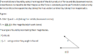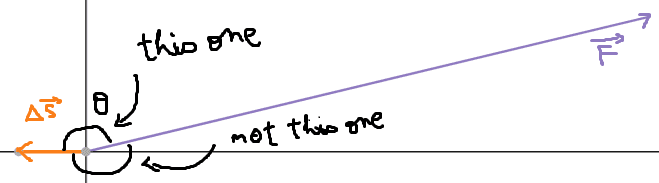# Work in two dimensions

Edel Crine
Homework Statement:
A force F=Fx ı +Fy j with Fx =50N and Fy =12N is exerted on a particle as the particle moves along the x axis from x=1.0m to x=-5.0m.
(a) Determine the work done by the force on the particle.
(b) What is the angle be- tween the force and the particle’s displacement?
Relevant Equations:
W=F*x
Since we don't know how far does it move in y-axis, I assumed that only x-component does work.
a) W=Fx*Δx = 50N*(-6m) = -300 J

b) Θ = arctan(12/50) = 13.5°

But, I'm not sure that did I do this in right way...h

Homework Helper
Gold Member
... as the particle moves along the x-axis from x=1.0m to x=-5.0m.
To clarify (in my mind,) is the particle constrained to "... move along the x- axis"?

because

"Since we don't know how far does it move in y-axis"
Well, is the particle constrained or not?

Edel Crine
To clarify (in my mind,) is the particle constrained to "... move along the x- axis"?

because

"Since we don't know how far does it move in y-axis"
Well, is the particle constrained or not?
Ummm it gave us only the displacement of x-axis, but we know the two components of the force, so I'm not sure whether does it move in y-direction as well, but I think so...?

Homework Helper
Gold Member
2022 Award
Ummm it gave us only the displacement of x-axis, but we know the two components of the force, so I'm not sure whether does it move in y-direction as well, but I think so...?
I think you are right to suppose it is constrained by some other force(s), so the motion is only in the x axis.
But think about the angle again. Draw a diagram showing the directions of force and displacement vectors.

Staff Emeritus
Homework Helper
Gold Member
Homework Statement:: A force F=Fx ı +Fy j with Fx =50N and Fy =12N is exerted on a particle as the particle moves along the x-axis from x=1.0m to x=-5.0m.
(a) Determine the work done by the force on the particle.
(b) What is the angle be- tween the force and the particle’s displacement?
Relevant Equations:: W=F*x

Since we don't know how far does it move in y-axis, I assumed that only x-component does work.
a) W=Fx*Δx = 50N*(-6m) = -300 J

b) Θ = arctan(12/50) = 13.5°

But, I'm not sure that did I do this in right way...h
My reading of the problem statement is that the particle literally moves along the x-axis. The y-coordinate there is zero. So its displacement has a y-component of zero.

By the way, I see that you used very nearly the same title for two threads you posted today. That's a bad idea.

Edel Crine
My reading of the problem statement is that the particle literally moves along the x-axis. The y-coordinate there is zero. So its displacement has a y-component of zero.

By the way, I see that you used the same title for two threads you posted today. That's a bad idea.
Oh yeah I did... 'Appreciate

Edel Crine
My reading of the problem statement is that the particle literally moves along the x-axis. The y-coordinate there is zero. So its displacement has a y-component of zero.

By the way, I see that you used very nearly the same title for two threads you posted today. That's a bad idea.
Then, I think I'm good for part a), but I'm not sure for part b)...

Edel Crine
I think you are right to suppose it is constrained by some other force(s), so the motion is only in the x axis.
But think about the angle again. Draw a diagram showing the directions of force and displacement vectors.
Ummm, would it be 0...?

Staff Emeritus
Homework Helper
Gold Member
Ummm, would it be 0...?
Are you guessing now?

Edel Crine
Are you guessing now?

I just used the x-component of the force and the displacement...
Let me try this again...

Edel Crine
Ummm I got 166°...?

Homework Helper
Gold Member
2022 Award
Ummm I got 166°...?
Yes.

Edel Crine
Yes.
Now, I see! I appreciate your help again...!

Bilbo B
Homework Statement:: A force F=Fx ı +Fy j with Fx =50N and Fy =12N is exerted on a particle as the particle moves along the x-axis from x=1.0m to x=-5.0m.
(a) Determine the work done by the force on the particle.
(b) What is the angle be- tween the force and the particle’s displacement?
Relevant Equations:: W=F*x

Since we don't know how far does it move in y-axis, I assumed that only x-component does work.
a) W=Fx*Δx = 50N*(-6m) = -300 J

b) Θ = arctan(12/50) = 13.5°

But, I'm not sure that did I do this in right way...h

#### Attachments

•Capture 1.PNG
6.6 KB · Views: 78
Homework Helper
Gold Member
2022 Award
(Image of a solution)
Your method for finding the work done is not effectively different from that in post #1.
For the angle, you have made the same mistake as in post #1.

archaic
Hello Edel!
From the way you wrote your response to a, I think that you have got the correct result just because the displacement was negative. I might be wrong, though.

For a constant force and a finite displacement with a constant direction, the work is defined as ##W=\vec F\cdot\Delta\vec s##, where ##\vec F## is the vector of the force for which you want to find the work, and ##\Delta\vec s## is the displacement vector.

We have, then, that ##W=|\vec F||\Delta\vec s|\cos\theta=(|\vec F|\cos\theta)|\Delta\vec s|=\overline{F_{||}}\times|\Delta\vec s|##.
##\theta## is the smallest angle you can make between the two vectors; If you draw two segments starting from the same point, then, starting from one vector, you can either rotate right or left to reach the other one.
##\overline{F_{||}}## represents the "signed" force in the direction of the displacement, i.e it can be positive or negative, depending ##\cos\theta##. (one can also leave force as it is and think of the "signed" component of the displacement in the direction of force, and it is more useful when the displacement's direction is variable while that of the force is constant.)
If the angle between your force vector and your displacement vector is not in ##(-\frac{\pi}{2},\,\frac{\pi}{2})##, then ##\overline{F_{||}}\leq0##. It is ##0## if ##\theta## takes either ##\pi/2## or ##-\pi/2##, because ##\cos\pm\frac{\pi}{2}=0##.

Let's go back to your problem (she has already solved it, don't delete my comment mods):$$\Delta\vec s=\vec x_f-\vec x_i=(-5.0-1.0)\hat\imath\\ \vec F=50\hat\imath+12\hat\jmath$$
You can see from the values of ##\vec F## that it points right from the up direction, and from the values of ##\Delta\vec s## that it points directly to the left direction. This tells us that the smallest angle between the two forces is obtuse, hence ##\overline{F_{||}}## is negative.
To calculate the work, just plug in the values: ##W=-|F_{||}||\Delta\vec s|=-1\times50\times6.0=-30.\times10^1\mathrm J##
To find the angle, also use the work formula, and I think that this was the aim of question b.
##W=|\vec F||\Delta\vec s|\cos\theta=-30.\times10^1##, thus ##\cos\theta=\frac{-30.\times10^1}{|\vec F||\Delta\vec s|}\Leftrightarrow\theta=\arccos\left(\frac{-30.\times10^1}{|\vec F||\Delta\vec s|}\right)\approx166.5^\circ\approx \left(17.\times10^1\right)^\circ##.
Sorry for the long post. 🤷‍♂️
For other usages, another definition of the dot product ##\vec u\cdot\vec v## is ##\left(u_xv_x+u_yv_y+u_zv_z+...\right)##. The ##+...## is for higher dimensions, you stop at what you have.

Last edited:
•Edel Crine